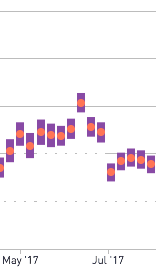NPSSome handy snippets for calculating NPS and accuracy.

[nps]

((promoters/total ) - (detractors/total))

[nps_var]

((1 - [NPS]) ^ 2 * promoters/total + (0 -  [NPS]) ^ 2 * neutral/total + (-1 - [NPS]) ^ 2 * detractors/total)

Suggested Usage:

select
npsdate
, total as sample_size
, [NPS] as nps
, (sqrt([NPS_VAR]) / sqrt(total))  as moe2
, (sqrt([NPS_VAR]) / sqrt(total))  as moe
, [NPS] - (sqrt([NPS_VAR]) / sqrt(total)) as moe_lower
from
(
select
[created:aggregation] as npsdate
, count(1)::float as total
, count(
case
when recommend <= 5
then 1
end )::float as detractors
, count(
case
when recommend > 5
and recommend < 9
then 1
end )::float as neutral
, count(
case
when recommend >= 9
then 1
end )::float as promoters
from
your_nps_survey_table
group by
1
)
as respondents

You can use moe_lower, moe and moe2 to show error bars on results. Make moe_lower the same color as the background.

7replies Oldest first
• Oldest first